# Colleges with the lowest SAT scores in Ohio

Top 10 colleges in Ohio with the lowest SAT scores
Looking for the colleges with the lowest SAT scores in Ohio? Well you're in luck! We've compiled a national college database and have created a list of the top 10 universities with the lowest SAT scores in Ohio below. If you are not a good test taker or worried about your test scores, this list is for you. These are the schools whose applicants had the lowest average SAT scores in Ohio, which means that you can get into these colleges with a lower SAT score. We also include each college's ACT scores and acceptance rate so that you can see where you would have the easiest time getting in. Read on to find out more.

## Central State University SAT scores

The average SAT score for Central State University is 800.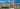The average SAT score of 800 breaks down into:

• SAT math: 390

The average ACT score for Central State University is 15 and their acceptance rate is 57.1%.

## Defiance College SAT scores

The average SAT score for Defiance College is 970.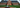The average SAT score of 970 breaks down into:

• SAT math: 490

The average ACT score for Defiance College is 20 and their acceptance rate is 56.5%.

## Tiffin University SAT scores

The average SAT score for Tiffin University is 1000.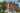The average SAT score of 1000 breaks down into:

• SAT math: 500

The average ACT score for Tiffin University is 20 and their acceptance rate is 69.2%.

## Urbana University SAT scores

The average SAT score for Urbana University is 1000.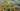The average SAT score of 1000 breaks down into:

• SAT math: 500

The average ACT score for Urbana University is 20 and their acceptance rate is 65%.

## Ohio Dominican University SAT scores

The average SAT score for Ohio Dominican University is 1025.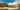The average SAT score of 1025 breaks down into:

• SAT math: 510

The average ACT score for Ohio Dominican University is 21 and their acceptance rate is 75.4%.

## Shawnee State University SAT scores

The average SAT score for Shawnee State University is 1040.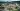The average SAT score of 1040 breaks down into:

• SAT math: 510

The average ACT score for Shawnee State University is 20 and their acceptance rate is 69.9%.

## Mount Saint Joseph University SAT scores

The average SAT score for Mount Saint Joseph University is 1060.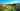The average SAT score of 1060 breaks down into:

• SAT math: 520

The average ACT score for Mount Saint Joseph University is 23 and their acceptance rate is 60.3%.

## Wilmington College SAT scores

The average SAT score for Wilmington College is 1060.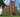The average SAT score of 1060 breaks down into:

• SAT math: 520

The average ACT score for Wilmington College is 21 and their acceptance rate is 84%.

## Kettering College SAT scores

The average SAT score for Kettering College is 1065.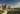The average SAT score of 1065 breaks down into:

• SAT math: 565

The average ACT score for Kettering College is 23 and their acceptance rate is 28%.

## John Carroll University SAT scores

The average SAT score for John Carroll University is 1066.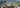The average SAT score of 1066 breaks down into:

• SAT math: 530